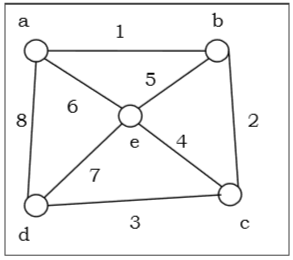# Depth First Search

Graph traversal is the problem of visiting all the vertices of a graph in some systematic order. There are mainly two ways to traverse a graph.

• Depth First Search

Depth First Search (DFS) algorithm starts from a vertex v, then it traverses to its adjacent vertex (say x) that has not been visited before and mark as "visited" and goes on with the adjacent vertex of x and so on.

If at any vertex, it encounters that all the adjacent vertices are visited, then it backtracks until it finds the first vertex having an adjacent vertex that has not been traversed before. Then, it traverses that vertex, continues with its adjacent vertices until it traverses all visited vertices and has to backtrack again. In this way, it will traverse all the vertices reachable from the initial vertex v.

DFS Algorithm

The concept is to visit all the neighbor vertices of a neighbor vertex before visiting the other neighbor vertices.

• Initialize status of all nodes as “Ready”

• Put source vertex in a stack and change its status to “Waiting”

• Repeat the following two steps until stack is empty −

• Pop the top vertex from the stack and mark it as “Visited”

• Push onto the top of the stack all neighbors of the removed vertex whose status is “Ready”. Mark their status as “Waiting”.

Problem

Let us take a graph (Source vertex is ‘a’) and apply the DFS algorithm to find out the traversal order.Solution

• Initialize status of all vertices to “Ready”.

• Push a in stack and change its status to “Waiting”.

• Pop a and mark it as “Visited”.

• Push a’s neighbors in “Ready” state e, d and b to top of stack and mark them as “Waiting”.

• Pop b from stack, mark it as “Visited”, push its “Ready” neighbor c onto stack.

• Pop c from stack and mark it as “Visited”. It has no “Ready” neighbor.

• Pop d from stack and mark it as “Visited”. It has no “Ready” neighbor.

• Pop e from stack and mark it as “Visited”. It has no “Ready” neighbor.

• Stack is empty. So stop.

So the traversal order is −

a → b → c → d → e

The alternate orders of traversal are −

a → e → b → c → d

Or, a → b → e → c → d

Or, a → d → e → b → c

Or, a → d → c → e → b

Or, a → d → c → b → e

Complexity Analysis

Let G(V, E) be a graph with |V| number of vertices and |E| number of edges. If DFS algorithm visits every vertex in the graph and checks every edge, then the time complexity is −

⊝( | V | + | E | )

Applications

• Detecting cycle in a graph
• To find topological sorting
• To test if a graph is bipartite
• Finding connected components
• Finding the bridges of a graph
• Finding bi-connectivity in graphs
• Solving the Knight’s Tour problem
• Solving puzzles with only one solution